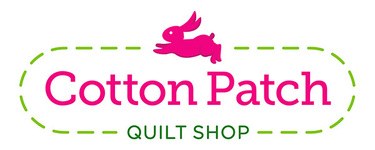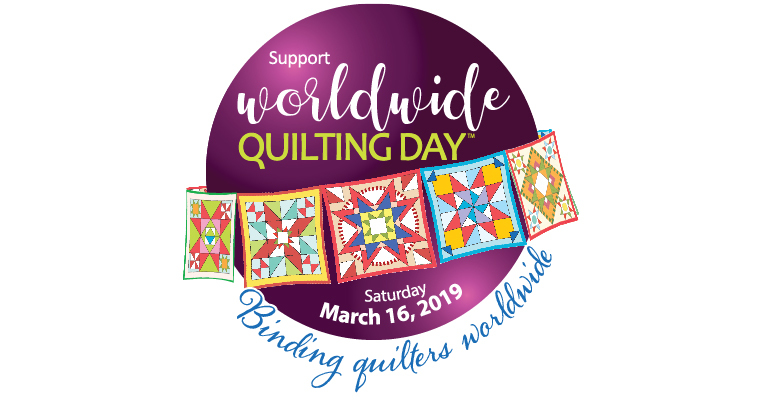# Worldwide Quilting Day!

March 16, 2019
Worldwide Quilting Day is the time each year that we celebrate quilting around the world and explore the ways that it binds us together.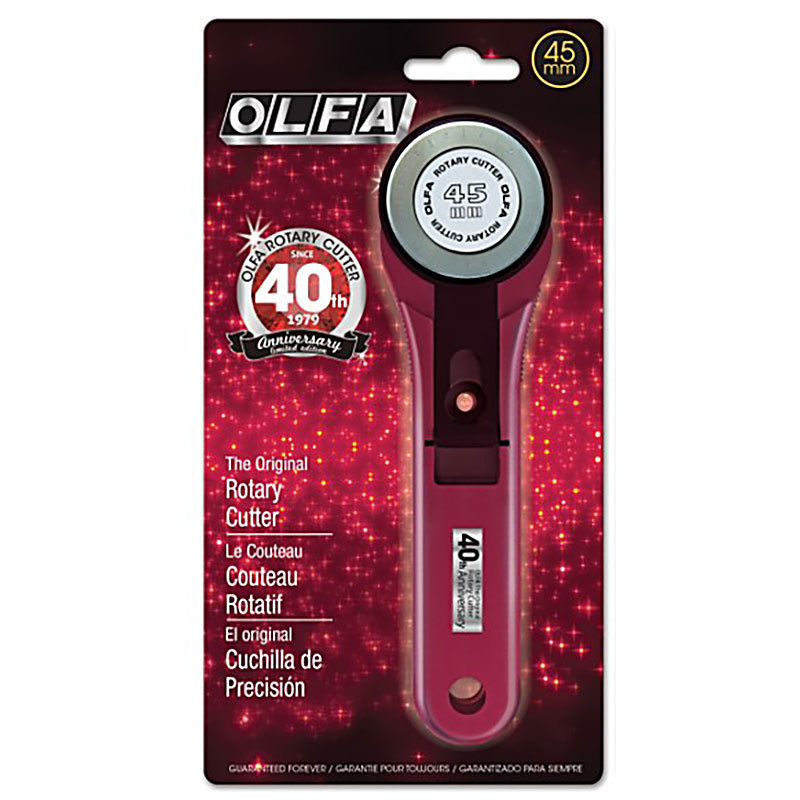Celebrate with us - we have soooo many new fabrics, patterns and notions for you to choose from!

## Saturday only

Spend \$100 or more and get a FREE Ruby Red Olfa Rotary Cutter!
Instore and Online

 table div table+table+table+table div table{width:100%;padding:0}table div table+table+table+table div table img{width:96.23%;padding:0;float:none}table div table+table+table+table div table td{width:100%;padding:0 1.88% 18px}/* styles */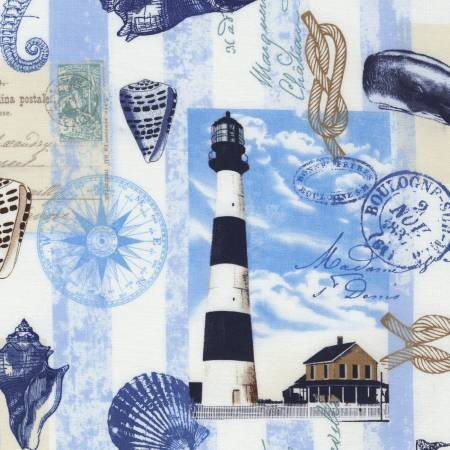# Nautical Mile

We know you love beachy and nautical prints and these new ones are awesome! They would make great beach bags and shirts as well as used in quilts.

 table div table+table+table+table+table+table+table div table{width:100%;padding:0}table div table+table+table+table+table+table+table div table img{width:96.23%;padding:0;float:none}table div table+table+table+table+table+table+table div table td{width:100%;padding:0 1.88% 18px}/* styles */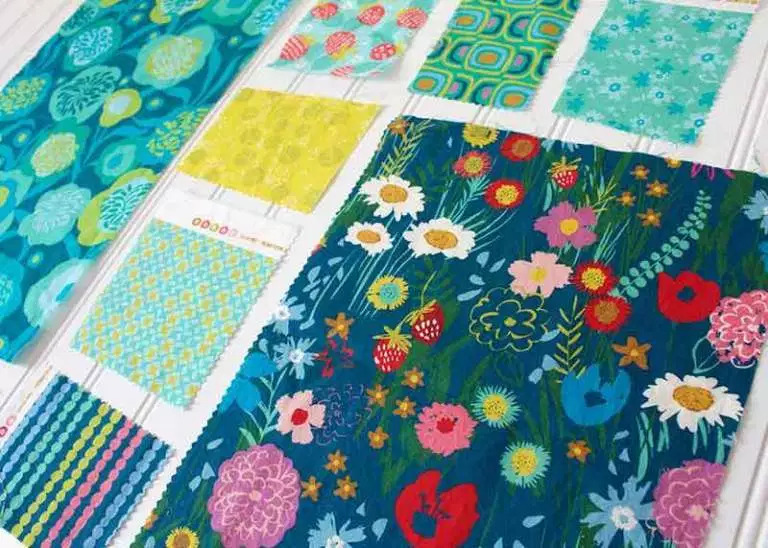# Growing Beautiful

New from Crystal Manning, Growing Beautiful is a vibrant colored collection filled with painterly flowers complemented with lively abstract patterns.
Also a rayon print, perfect for making garments!

 table div table+table+table+table+table+table+table+table+table+table div table{width:100%;padding:0}table div table+table+table+table+table+table+table+table+table+table div table img{width:96.23%;padding:0;float:none}table div table+table+table+table+table+table+table+table+table+table div table td{width:100%;padding:0 1.88% 18px}/* styles */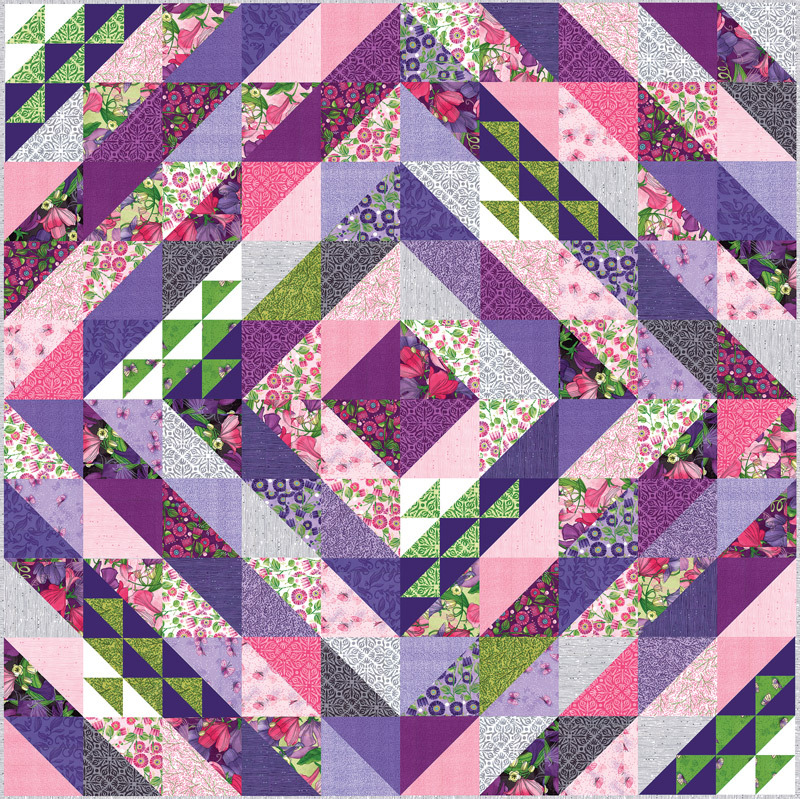# Attention Purple Fans!

You are going to love this new Moda line!
Sweet Pea & Lily combines purples, greens, pinks and grays in a mix of sweetness and royalty. The purple hues range from cool to warm shades. There is something dramatic about the mixture and play of deep purple with lavender and the warm of pinks and the lively plum

 /* styles */ We have kits for this stunning quilt that come packaged in a darling box - and we are offering an introductory price from 3/14 through 3/17. Regular price \$134.99, intro price just \$120. Limited to quantities on hand, so don't wait. My sample hasn't been quilted yet, but you can see how gorgeous and BIG this quilt is!
 table div table+table+table+table+table+table+table+table+table+table+table+table+table+table div table{width:100%;padding:0}table div table+table+table+table+table+table+table+table+table+table+table+table+table+table div table img{width:96.23%;padding:0;float:none}table div table+table+table+table+table+table+table+table+table+table+table+table+table+table div table td{width:100%;padding:0 1.88% 18px}/* styles */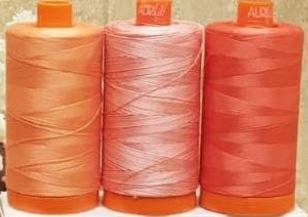# Living Coral Threads

Pantone declared Living Coral as the color of the year.
Aurifil curated this limited edition package of coral threads in medium, dark and variegated, 50 weight.

 table div table+table+table+table+table+table+table+table+table+table+table+table+table+table+table+table+table div table{width:100%;padding:0}table div table+table+table+table+table+table+table+table+table+table+table+table+table+table+table+table+table div table img{width:96.23%;padding:0;float:none}table div table+table+table+table+table+table+table+table+table+table+table+table+table+table+table+table+table div table td{width:100%;padding:0 1.88% 18px}/* styles */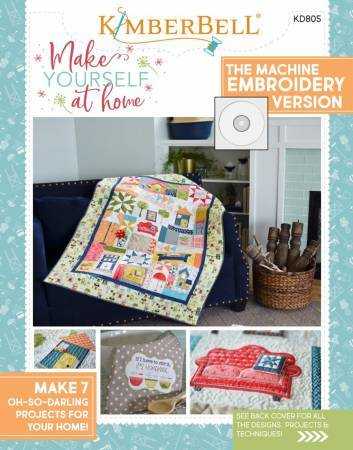# Make Yourself at Home by Kimberbell!

Whether you live in a free standing house, an apartment building or a camper, there are cute motifs to customize your project.
There is the big quilt and 6 bonus projects in this Machine Embroidery version. Love the sofa with the dimensional pillow!

 table div table+table+table+table+table+table+table+table+table+table+table+table+table+table+table+table+table+table+table+table div table{width:100%;padding:0}table div table+table+table+table+table+table+table+table+table+table+table+table+table+table+table+table+table+table+table+table div table img{width:96.23%;padding:0;float:none}table div table+table+table+table+table+table+table+table+table+table+table+table+table+table+table+table+table+table+table+table div table td{width:100%;padding:0 1.88% 18px}/* styles */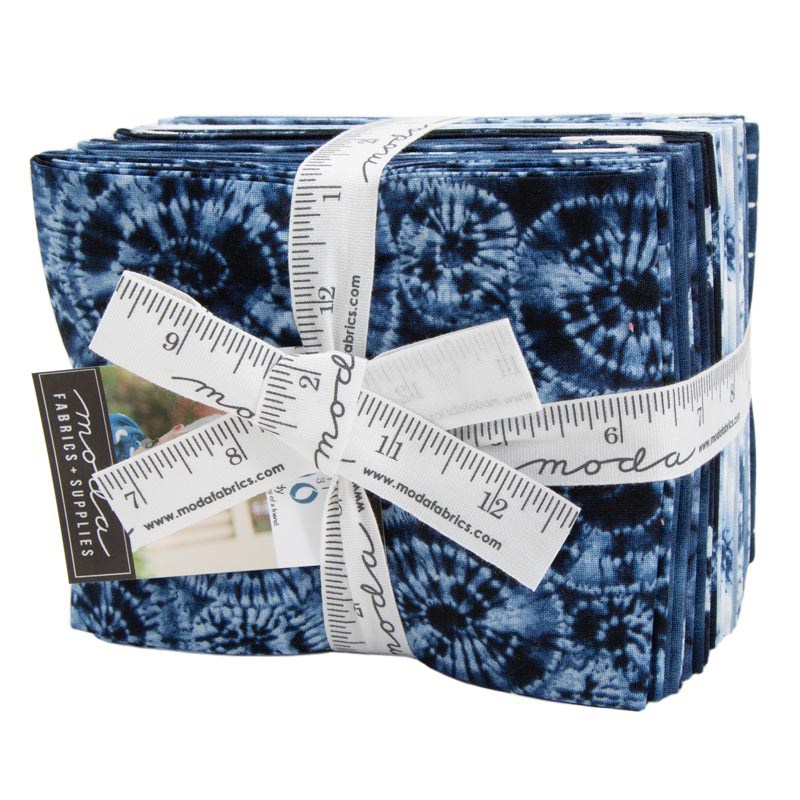# Fat Quarter Bundles

We added a few new, full collection fat quarter bundles this week. We only have 1 of each bundle so don't dally!

 table div table+table+table+table+table+table+table+table+table+table+table+table+table+table+table+table+table+table+table+table+table+table+table div table{width:100%;padding:0}table div table+table+table+table+table+table+table+table+table+table+table+table+table+table+table+table+table+table+table+table+table+table+table div table img{width:96.23%;padding:0;float:none}table div table+table+table+table+table+table+table+table+table+table+table+table+table+table+table+table+table+table+table+table+table+table+table div table td{width:100%;padding:0 1.88% 18px}/* styles */## Stitch Therapy 365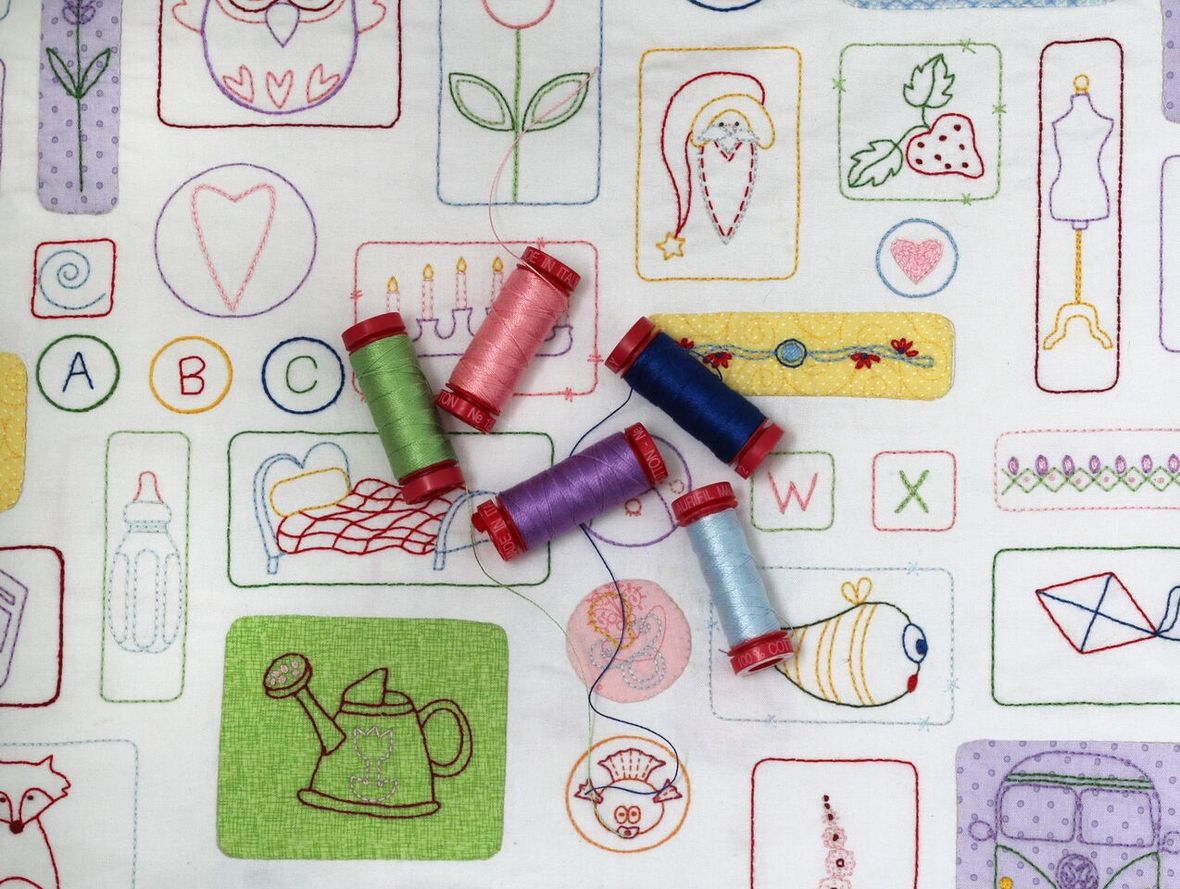It's not too late!
We have panels and thread kits AND...
your 365 days start when you get signed up, so no playing catch up, just a little stitching every day.

 table div table+table+table+table+table+table+table+table+table+table+table+table+table+table+table+table+table+table+table+table+table+table+table+table+table+table div table{width:100%;padding:0}table div table+table+table+table+table+table+table+table+table+table+table+table+table+table+table+table+table+table+table+table+table+table+table+table+table+table div table img{width:96.23%;padding:0;float:none}table div table+table+table+table+table+table+table+table+table+table+table+table+table+table+table+table+table+table+table+table+table+table+table+table+table+table div table td{width:100%;padding:0 1.88% 18px}/* styles */# Next PMS Night is Friday, March 15

6pm to midnight
Treat yourself to a night of food, fabric and friends!

 table div table+table+table+table+table+table+table+table+table+table+table+table+table+table+table+table+table+table+table+table+table+table+table+table+table+table+table+table+table div table{width:100%;padding:0}table div table+table+table+table+table+table+table+table+table+table+table+table+table+table+table+table+table+table+table+table+table+table+table+table+table+table+table+table+table div table img{width:96.23%;padding:0;float:none}table div table+table+table+table+table+table+table+table+table+table+table+table+table+table+table+table+table+table+table+table+table+table+table+table+table+table+table+table+table div table td{width:100%;padding:0 1.88% 18px}/* styles */# Casual Sew 10am to 4pm

Friday, March 15, 22, 29

# Upcoming Classes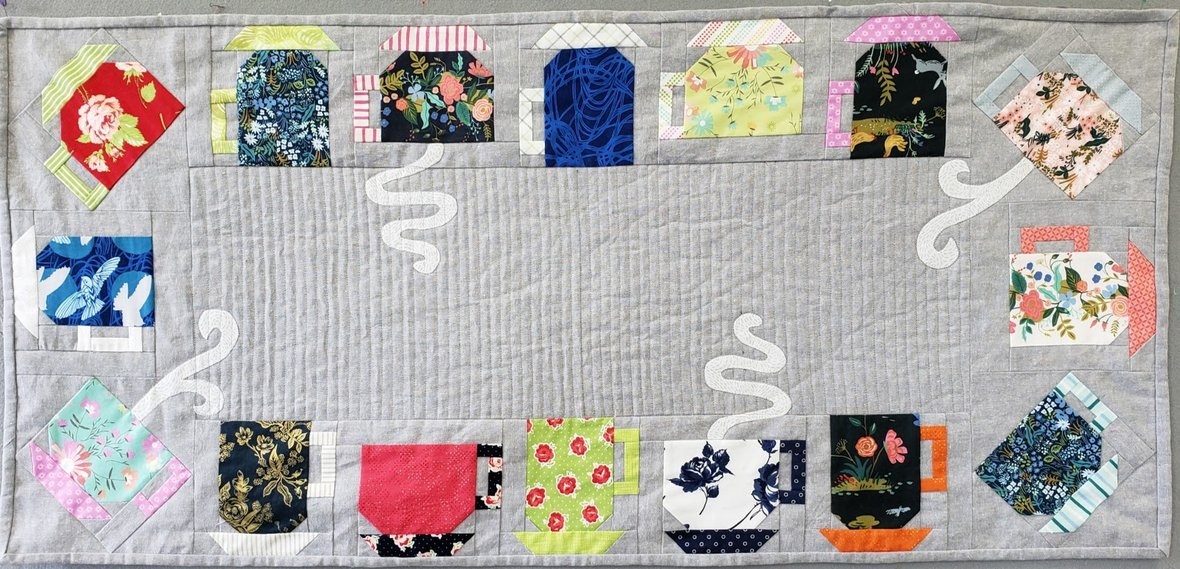# SEW CAFFEINATED

Another table runner featured in Trendy Table 2!
This is a fun table runner to make with colorful cups and saucers.
Some of the cups are tall like mugs and others are short and wider
Class is Saturday, March 16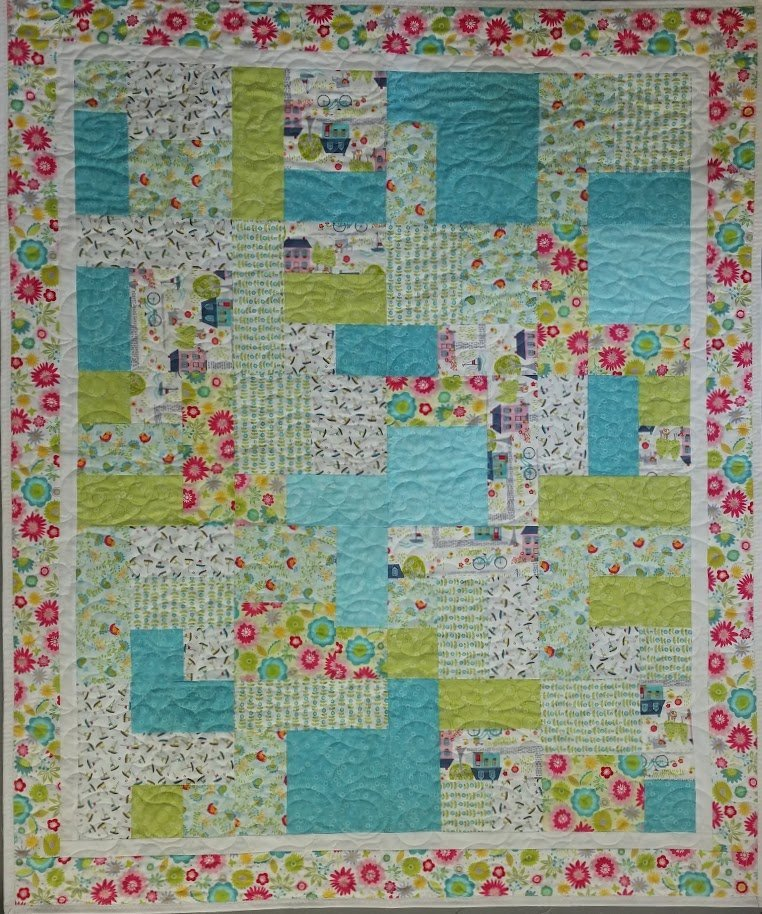# Yellow Brick Road

A classic quilt pattern that every quilter should make at least once!
3 part class is paced for newer quilters.
3 Sundays, beginning March 17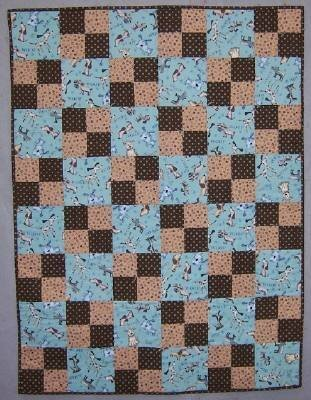# Beginning Quilt Making

Class starts Tuesday, March 19
Day or evening sessions available

## One spot left in each!

If you always wanted to make a quilt, this class is for you!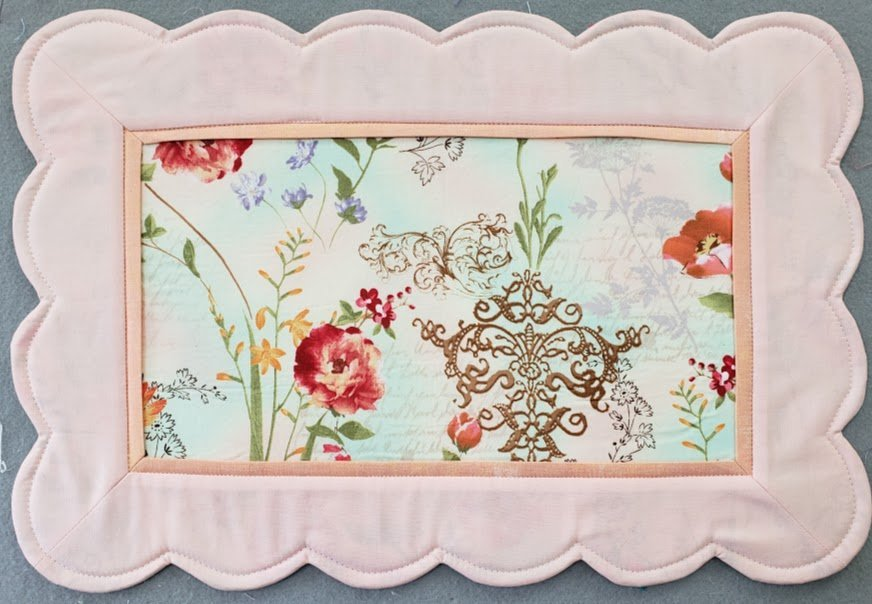# Scalloped Placemats

Set a pretty table with these reversible scalloped placemats!
Class is Monday, March 18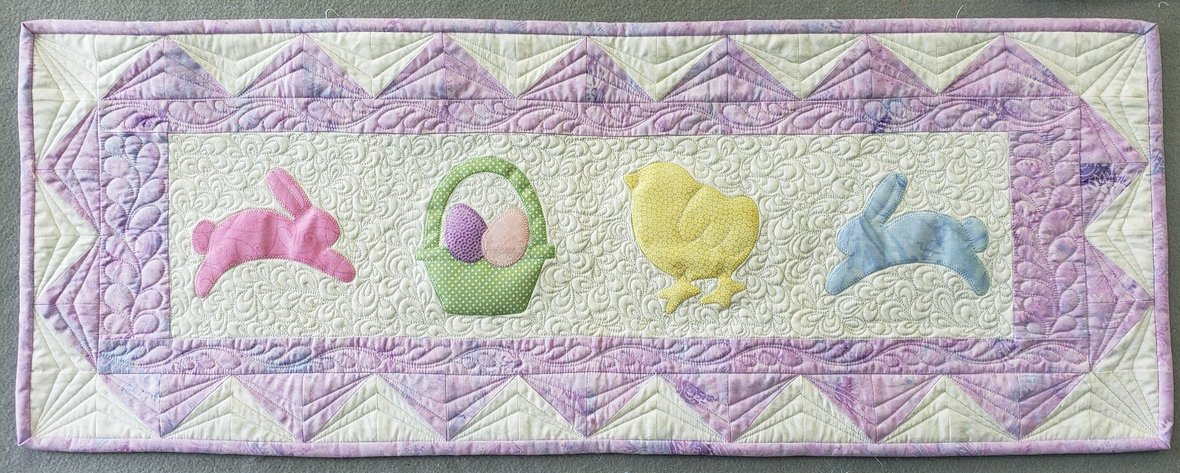# GO! Spring Medley Table Runner

Join Pat to make a cute Spring table runner using the new die from Accuquilt
Class is Thursday, March 21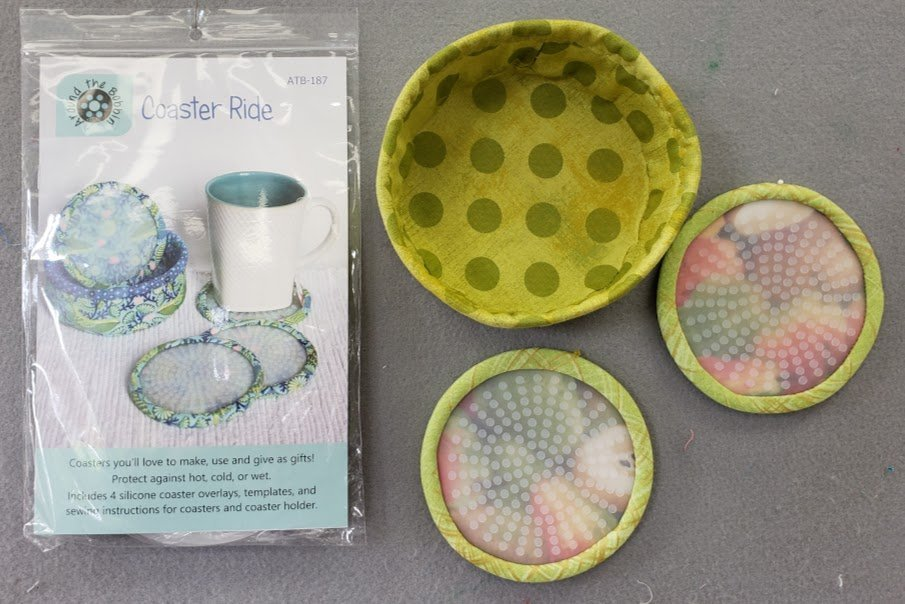Coaster Ride
An ideal project for your home or for gifts!
Class is Saturday, March 23

# And there's more!

 table div table+table+table+table+table+table+table+table+table+table+table+table+table+table+table+table+table+table+table+table+table+table+table+table+table+table+table+table+table+table+table+table+table+table+table+table+table+table+table+table+table+table+table+table div table{width:100%;padding:0}table div table+table+table+table+table+table+table+table+table+table+table+table+table+table+table+table+table+table+table+table+table+table+table+table+table+table+table+table+table+table+table+table+table+table+table+table+table+table+table+table+table+table+table+table div table img{width:96.23%;padding:0;float:none}table div table+table+table+table+table+table+table+table+table+table+table+table+table+table+table+table+table+table+table+table+table+table+table+table+table+table+table+table+table+table+table+table+table+table+table+table+table+table+table+table+table+table+table+table div table td{width:100%;padding:0 1.88% 18px}/* styles */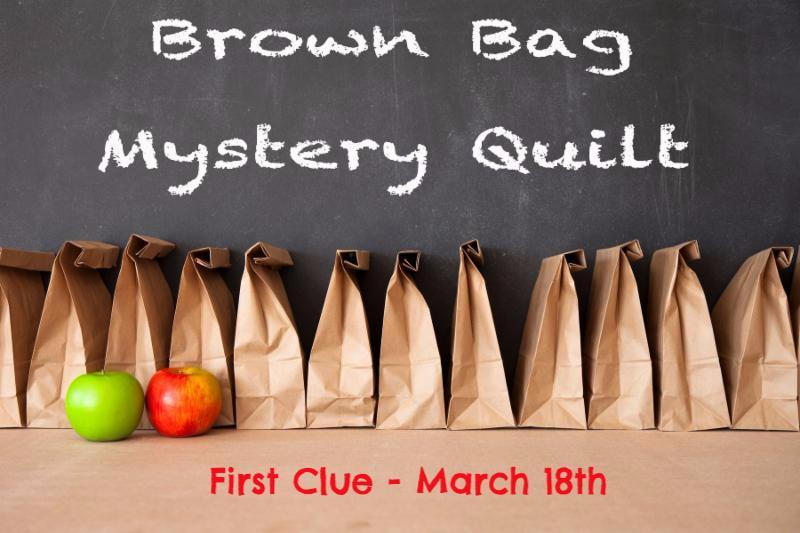# Brown Bag Mystery!

The first clue will be ready this Saturday, March 16 and it's not too late to get a bag!
We have a great assortment to choose from, Batiks in several colorways, mermaids and more!

If you joined us last year, you know what fun it is!
It's a true mystery - you don't know what the pattern is and you don't know what all the fabrics are!
The bow on the bag is your only "clue" - if you like that fabric, you will like your fabrics.

And, yes, you can play even if you live far away!

## ps, the Creative Grids tool that the designer recommended is in stock now! Click on the link below.

 table div table+table+table+table+table+table+table+table+table+table+table+table+table+table+table+table+table+table+table+table+table+table+table+table+table+table+table+table+table+table+table+table+table+table+table+table+table+table+table+table+table+table+table+table+table+table+table+table div table{width:100%;padding:0}table div table+table+table+table+table+table+table+table+table+table+table+table+table+table+table+table+table+table+table+table+table+table+table+table+table+table+table+table+table+table+table+table+table+table+table+table+table+table+table+table+table+table+table+table+table+table+table+table div table img{width:96.23%;padding:0;float:none}table div table+table+table+table+table+table+table+table+table+table+table+table+table+table+table+table+table+table+table+table+table+table+table+table+table+table+table+table+table+table+table+table+table+table+table+table+table+table+table+table+table+table+table+table+table+table+table+table div table td{width:100%;padding:0 1.88% 18px}/* styles */# Open 7 days a week for your convenience!

Monday through Saturday, 10am to 5pm
Sunday 12pm to 4pm

See you soon!

Happy St Patrick's Day from Lucky McWiggles!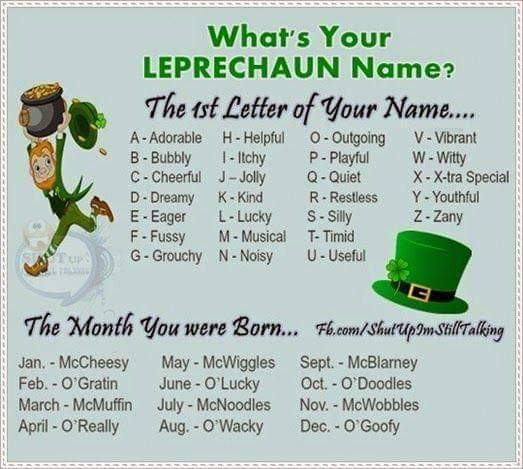table div table+table+table+table+table+table+table+table+table+table+table+table+table+table+table+table+table+table+table+table+table+table+table+table+table+table+table+table+table+table+table+table+table+table+table+table+table+table+table+table+table+table+table+table+table+table+table+table+table+table+table div table{width:100%;padding:0}table div table+table+table+table+table+table+table+table+table+table+table+table+table+table+table+table+table+table+table+table+table+table+table+table+table+table+table+table+table+table+table+table+table+table+table+table+table+table+table+table+table+table+table+table+table+table+table+table+table+table+table div table img{width:96.23%;padding:0;float:none}table div table+table+table+table+table+table+table+table+table+table+table+table+table+table+table+table+table+table+table+table+table+table+table+table+table+table+table+table+table+table+table+table+table+table+table+table+table+table+table+table+table+table+table+table+table+table+table+table+table+table+table div table td{width:100%;padding:0 1.88% 18px}/* styles *//* styles */ Freddie is just chillin'
 table.module-52{width:66.04%;padding:0}table div table+table+table+table+table+table+table+table+table+table+table+table+table+table+table+table+table+table+table+table+table+table+table+table+table+table+table+table+table+table+table+table+table+table+table+table+table+table+table+table+table+table+table+table+table+table+table+table+table+table+table+table+table div table{width:66.04%;float:none;margin-left:auto;margin-right:auto;padding:0}table div table+table+table+table+table+table+table+table+table+table+table+table+table+table+table+table+table+table+table+table+table+table+table+table+table+table+table+table+table+table+table+table+table+table+table+table+table+table+table+table+table+table+table+table+table+table+table+table+table+table+table+table+table div table a{border:0 none;text-decoration:none}table div table+table+table+table+table+table+table+table+table+table+table+table+table+table+table+table+table+table+table+table+table+table+table+table+table+table+table+table+table+table+table+table+table+table+table+table+table+table+table+table+table+table+table+table+table+table+table+table+table+table+table+table+table div table img{width:100%!important;border:0 none;text-decoration:none}table div table+table+table+table+table+table+table+table+table+table+table+table+table+table+table+table+table+table+table+table+table+table+table+table+table+table+table+table+table+table+table+table+table+table+table+table+table+table+table+table+table+table+table+table+table+table+table+table+table+table+table+table+table div table td{width:100%;padding:0}/* styles */
 Like   Pin   +1